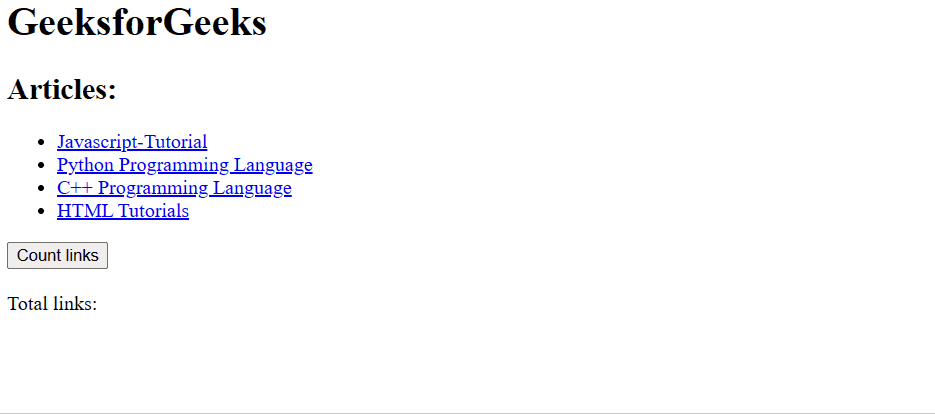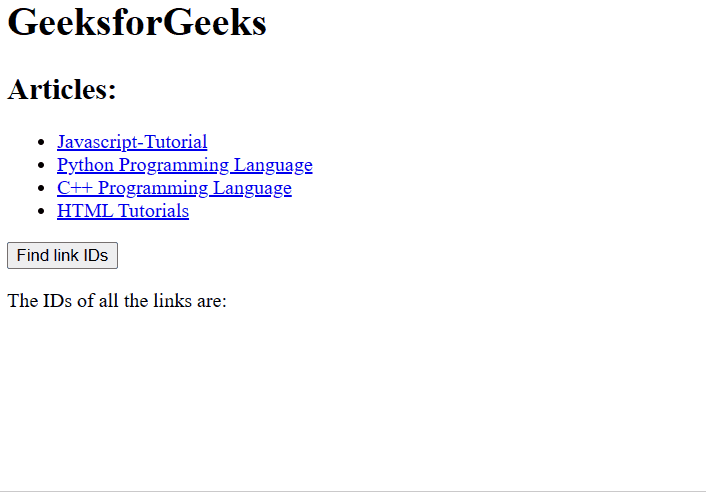Open In App

The DOM links collection is used to return the collection of all <a> and <area> elements with the “href” attribute in the HTML document. The elements in the collection are sorted as they appear in the sourcecode.

Syntax:

`document.links`

Properties: It contains a single property length which is used to return the number of <a> and <area> elements in the collection.

Methods: The DOM links collection contains three methods which are listed below:

• [index]: It is used to return the <a> and <area> element of the specified index. The index value starts with 0. The NULL value is returned if the index value is out of range.
• item(index): It is used to return the <a> and <area> element of selected index. The index value starts with 0. The NULL value is returned if the index value is out of range. This method performs similarly to the above method.
• namedItem(id): It is used to return the <a> and <area> element from the collection which matches the specified id. NULL is returned if the id does not exist.

Return Value: An HTMLCollection Object, representing all <a> elements and/or <area> elements in the document. The elements in the collection are sorted as they appear in the source code

The below programs illustrate the use of the documents.links property in HTML:

Example 1: Using the length property to count the number of link elements in the collection.

## HTML

 ```<``html``>``<``head``>``    ``<``title``>``        ``DOM document.links() Property``    ````    ````    ``<``script``>``        ``function countLinks() {``            ``let collection = document.links.length;``            ``document.querySelector(".count").innerHTML =``                ``collection;``        ``}``    ``````<``body``>``    ``<``h1``>GeeksforGeeks``    ``<``h2``>Articles:``    ``<``ul``>``        ````        ``<``li``>``            ``<``a` `id``=``"js"``               ``href``=``"https://www.geeksforgeeks.org/javascript-tutorial/"``>``                ``Javascript-Tutorial``            ````        ````        ``<``li``>``            ``<``a` `id``=``"python"``               ``href``=``"https://www.geeksforgeeks.org/python-programming-language/"``>``                ``Python Programming Language``            ````        ````        ``<``li``>``            ``<``a` `id``=``"cpp"``               ``href``=``"https://www.geeksforgeeks.org/c-plus-plus/"``>``                ``C++ Programming Language``            ````        ````        ``<``li``>``            ``<``a` `id``=``"html"``               ``href``=``"https://www.geeksforgeeks.org/html-tutorials/"``>``                ``HTML Tutorials``            ````        ````    ````    ````    ``<``button` `onclick``=``"countLinks()"``>``        ``Count links``    ````    ``<``br``>``    ``<``br``>``    ``<``span``>Total links: ``    ``<``span` `class``=``"count"``>`````

Output:Example 2: HTML code to find all links in the document and return their IDs.

## HTML

 ```<``html``>``<``head``>``    ``<``title``>``        ``DOM document.links() Property``    ``` `    ``<``script``>``        ``/* function to find IDs */``        ``function findLinkIDs() {``            ``let final = '';``            ``let collection = document.links;``            ``// Run a for loop upto the number of``            ``// links in the collection``            ``for (let i = 0; i < ``collection.length``; i++) {``                ``// Add each link id to a list``                ``final += `
• \${collection[i].id} `;``            ``}``            ``// Replace the HTML of the ID div``            ``document.querySelector(".ids").innerHTML =``                ``final;``        ``}``    ``````<``body``>``    ``<``h1``>GeeksforGeeks``    ``<``h2``>Articles:``    ``<``ul``>``        ````        ``<``li``>``            ``<``a` `id``=``"js"``               ``href``=``"https://www.geeksforgeeks.org/javascript-tutorial/"``>``                ``Javascript-Tutorial``            ````        ````        ``<``li``>``            ``<``a` `id``=``"python"``               ``href``=``"https://www.geeksforgeeks.org/python-programming-language/"``>``                ``Python Programming Language``            ````        ````        ``<``li``>``            ``<``a` `id``=``"cpp"``               ``href``=``"https://www.geeksforgeeks.org/c-plus-plus/"``>``                ``C++ Programming Language``            ````        ````        ``<``li``>``            ``<``a` `id``=``"html"``               ``href``=``"https://www.geeksforgeeks.org/html-tutorials/"``>``                ``HTML Tutorials``            ````        ````    ````    ````    ``<``button` `onclick``=``"findLinkIDs()"``>``        ``Find link IDs``    ````    ``<``p``>The IDs of all the links are: ``    ``<``div` `class``=``"ids"``>`````
• Output:Example 3: Using the id property to find by link ID and display its href attribute

## html

 ```<``html``>``<``head``>``    ``<``title``>``        ``DOM document.links() Property``    ````    ``<``script``>``        ``function returnLinkByID() {``            ``let collection =``                ``document.links.namedItem("js");``            ``// Get the href attribute``            ``let link = collection.href;``            ``document.querySelector(".name").innerHTML =``                ``link;``        ``}``    ````` `<``body``>``    ``<``h1``>GeeksforGeeks``    ``<``h2``>Articles:``    ``<``ul``>``        ````        ``<``li``>``            ``<``a` `id``=``"js"``               ``href``=``"https://www.geeksforgeeks.org/javascript-tutorial/"``>``                ``Javascript-Tutorial``            ````        ````        ``<``li``>``            ``<``a` `id``=``"python"``               ``href``=``"https://www.geeksforgeeks.org/python-programming-language/"``>``                ``Python Programming Language``            ````        ````        ``<``li``>``            ``<``a` `id``=``"cpp"``               ``href``=``"https://www.geeksforgeeks.org/c-plus-plus/"``>``                ``C++ Programming Language``            ````        ````        ``<``li``>``            ``<``a` `id``=``"html"``               ``href``=``"https://www.geeksforgeeks.org/html-tutorials/"``>``                ``HTML Tutorials``            ````        ````    ````    ``<``button` `onclick``=``"returnLinkByID()"``>``        ``Find by link ID``    ````    ``<``p``>``        ``The href attribute in the link``        ``with id of 'js' is:``    ````    ``<``div` `class``=``"name"``>`````

Output:Supported Browsers: The browser supported by DOM links collection method are listed below:

• Chrome 1 and above
• Edge 12 and above
• Internet Explorer 4 and above
• Firefox 1 and above
• Opera 12.1 and above
• Safari 1 and above